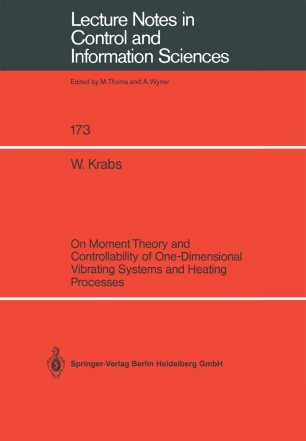# On Moment Theory and Controllability of One-Dimensional Vibrating Systems and Heating Processes

• Editors
• Werner KrabsBook

Part of the Lecture Notes in Control and Information Sciences book series (LNCIS, volume 173)

1. Front Matter
2. Pages 26-116
3. Pages 117-168
4. Back Matter

### Introduction

The main concern of this book is the application of infinite moment theory to the problem of controllability of one-di- mensional vibrating systems (like strings and beams) and heating processes. Distributed as well as boundary control is considered. In the case of vibrating systems trigonometric moment pro- blems are to be investigated which is done on the basis of an abstract moment theory in Hilbert spaces. Equivalently, alsothe theory of linear operator equations on Hilbert spa- ces ( partly with unbounded operators) is applied to the problem of controllability and time-minimal controllability. In the case of heating processes exponential moment problems are to be dealt with which is done on the basis of an ab- stract moment theory in Banach spaces. Time-minimal control- lability is also treated with the aid of the theory of line- aroperator equations on Banach spaces. Some advanced knowledge in functional analyis and on partial differential equations is preassumed for a fluent reader of the book, but parts of it are also readable with basic ma- thematical knowledge (for instance, the finite-dimensional part of the introduction).

### Keywords

Controlliability Heat Diffusion Linear Vibrations Lineare Vibration Moment Problems Operator Equations Regelung control control system damping differential equation operator optimal control partial differential equation vibration

### Bibliographic information

• DOI https://doi.org/10.1007/BFb0039513